# Truncated icosahedron

Truncated icosahedron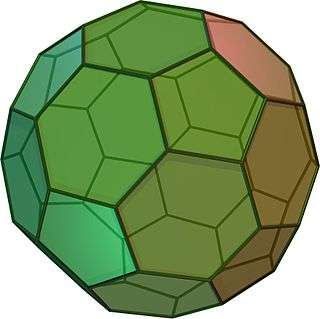TypeArchimedean solid
Uniform polyhedron
ElementsF = 32, E = 90, V = 60 (χ = 2)
Faces by sides12{5}+20{6}
Conway notationtI
Schläfli symbolst{3,5}
t0,1{3,5}
Wythoff symbol2 5 | 3
Coxeter diagramSymmetry groupIh, H3, [5,3], (*532), order 120
Rotation groupI, [5,3]+, (532), order 60
Dihedral Angle6-6: 138.189685°
6-5: 142.62°
ReferencesU25, C27, W9
PropertiesSemiregular convex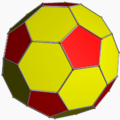Colored faces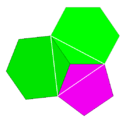5.6.6
(Vertex figure)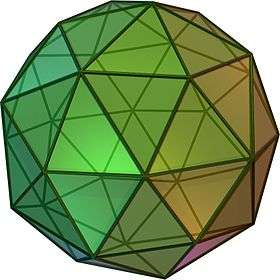Pentakis dodecahedron
(dual polyhedron)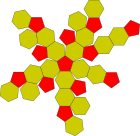Net

In geometry, the truncated icosahedron is an Archimedean solid, one of 13 convex isogonal nonprismatic solids whose faces are two or more types of regular polygons.

It has 12 regular pentagonal faces, 20 regular hexagonal faces, 60 vertices and 90 edges.

It is the Goldberg polyhedron GV(1,1), containing pentagonal and hexagonal faces.

This geometry is associated with footballs (soccer balls) typically patterned with white hexagons and black pentagons. Geodesic domes such as those whose architecture Buckminster Fuller pioneered are often based on this structure. It also corresponds to the geometry of the fullerene C60 ("buckyball") molecule.

It is used in the cell-transitive hyperbolic space-filling tessellation, the bitruncated order-5 dodecahedral honeycomb.

## Construction

This polyhedron can be constructed from an icosahedron with the 12 vertices truncated (cut off) such that one third of each edge is cut off at each of both ends. This creates 12 new pentagon faces, and leaves the original 20 triangle faces as regular hexagons. Thus the length of the edges is one third of that of the original edges.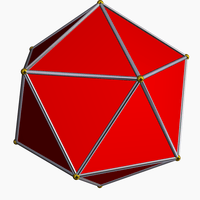Icosahedron

## Cartesian coordinates

Cartesian coordinates for the vertices of a truncated icosahedron centered at the origin are all even permutations of:

(0, ±1, ±3φ)
(±2, ±(1 + 2φ), ±φ)
(±1, ±(2 + φ), ±2φ)

where φ = 1 + 5/2 is the golden mean. Using φ2 = φ + 1 one verifies that all vertices are on a sphere, centered at the origin, with the radius equal to 9φ + 10. The edges have length 2.

Permutations:

X axis
(±3φ, 0, ±1)
(±(1 + 2φ), ±φ, ±2)
(±(2 + φ), ±2φ, ±1)
Y axis
(±1, ±3φ, 0)
(±2, ±(1 + 2φ), ±φ)
(±1, ±(2 + φ), ±2φ)
Z axis
(0, ±1, ±3φ)
(±φ, ±2, ±(1 + 2φ))
(±2φ, ±1, ±(2 + φ))

## Orthogonal projections

The truncated icosahedron has five special orthogonal projections, centered, on a vertex, on two types of edges, and two types of faces: hexagonal and pentagonal. The last two correspond to the A2 and H2 Coxeter planes.

Orthogonal projections
Centered by Vertex Edge
5-6
Edge
6-6
Face
Hexagon
Face
Pentagon
Image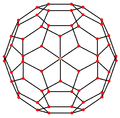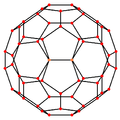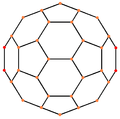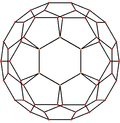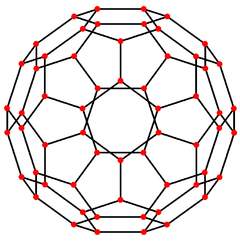Projective
symmetry
    
Dual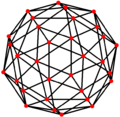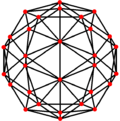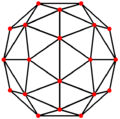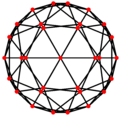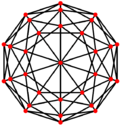## Spherical tiling

The truncated icosahedron can also be represented as a spherical tiling, and projected onto the plane via a stereographic projection. This projection is conformal, preserving angles but not areas or lengths. Straight lines on the sphere are projected as circular arcs on the plane.

 Orthographic projection Stereographic projections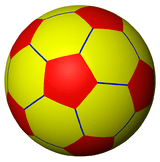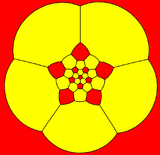pentagon-centered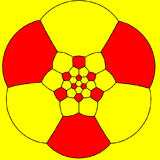hexagon-centered

## Dimensions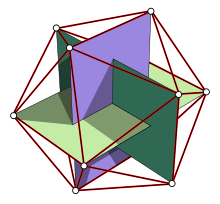Mutually orthogonal golden rectangles drawn into the original icosahedron (before cut off)

If the edge length of a truncated icosahedron is a, the radius of a circumscribed sphere (one that touches the truncated icosahedron at all vertices) is:where φ is the golden ratio.

This result is easy to get by using one of the three orthogonal golden rectangles drawn into the original icosahedron (before cut off) as the starting point for our considerations. The angle between the segments joining the center and the vertices connected by shared edge (calculated on the basis of this construction) is approximately 23.281446°.

## Area and volume

The area A and the volume V of the truncated icosahedron of edge length a are:## Geometric relations

The truncated icosahedron easily demonstrates the Euler characteristic:

32 + 60 − 90 = 2.

With unit edges, the surface area is (rounded) 21 for the pentagons and 52 for the hexagons, together 73 (see areas of regular polygons).

## Applications

The balls used in association football and team handball are perhaps the best-known example of a spherical polyhedron analog to the truncated icosahedron, found in everyday life. The ball comprises the same pattern of regular pentagons and regular hexagons, but it is more spherical due to the pressure of the air inside and the elasticity of the ball. This ball type was introduced to the World Cup in 1970 (starting in 2006, this iconic design has been superseded by alternative patterns).

Geodesic domes are typically based on triangular facetings of this geometry with example structures found across the world, popularized by Buckminster Fuller.

A variation of the icosahedron was used as the basis of the honeycomb wheels (made from a polycast material) used by the Pontiac Motor Division between 1971 and 1976 on its Trans Am and Grand Prix.

This shape was also the configuration of the lenses used for focusing the explosive shock waves of the detonators in both the gadget and Fat Man atomic bombs.

The truncated icosahedron can also be described as a model of the Buckminsterfullerene (fullerene) (C60), or "buckyball," molecule, an allotrope of elemental carbon, discovered in 1985. The diameter of the football and the fullerene molecule are 22 cm and about 1 nm, respectively, hence the size ratio is 220,000,000:1.

## Truncated icosahedra in the arts

A truncated icosahedron with "solid edges" by Leonardo da Vinci appears as an illustration in Luca Pacioli's book De divina proportione.

These uniform star-polyhedra, and one icosahedral stellation have nonuniform truncated icosahedra convex hulls:

## Truncated icosahedral graph

Truncated icosahedral graph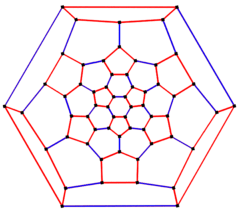6-fold symmetry schlegel diagram
Vertices 60
Edges 90
Automorphisms 120
Chromatic number 2
Properties Cubic, Hamiltonian, regular, zero-symmetric

In the mathematical field of graph theory, a truncated icosahedral graph is the graph of vertices and edges of the truncated icosahedron, one of the Archimedean solids. It has 60 vertices and 90 edges, and is a cubic Archimedean graph.5-fold symmetry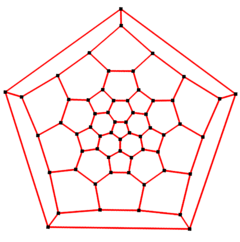5-fold Schlegel diagramLook up truncated icosahedron in Wiktionary, the free dictionary.